upm  1.7.1 Sensor/Actuator repository for libmraa (v2.0.0)
MPU60X0 Class Reference

API for the MPU60X0 3-axis Gyroscope and 3-axis Accelerometer. More...

## Detailed Description

The MPU60X0 devices provide the world's first integrated 6-axis motion processor solution that eliminates the package-level gyroscope and accelerometer cross-axis misalignment associated with discrete solutions. The devices combine a 3-axis gyroscope and a 3-axis accelerometer on the same silicon die.

While not all of the functionality of this device is supported initially, methods and register definitions are provided that should allow an end user to implement whatever features are required.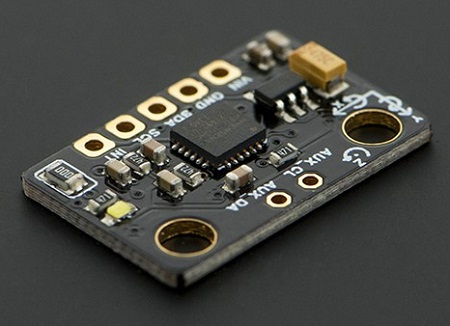upm::MPU60X0 sensor;
sensor.init();
while (shouldRun) {
sensor.update();
float x, y, z;
sensor.getAccelerometer(&x, &y, &z);
cout << "Accelerometer: ";
cout << "AX: " << x << " AY: " << y << " AZ: " << z << endl;
sensor.getGyroscope(&x, &y, &z);
cout << "Gryoscope: ";
cout << "GX: " << x << " GY: " << y << " GZ: " << z << endl;
cout << "Temperature: " << sensor.getTemperature() << endl;
cout << endl;
upm_delay_us(500000);
}

## Public Types

enum  MPU60X0_REG_T {
REG_SELF_TEST_X = 0x0d, REG_SELF_TEST_Y = 0x0e, REG_SELF_TEST_Z = 0x0f, REG_SELF_TEST_A = 0x10,
REG_SMPLRT_DIV = 0x19, REG_CONFIG = 0x1a, REG_GYRO_CONFIG = 0x1b, REG_ACCEL_CONFIG = 0x1c,
REG_FF_THR = 0x1d, REG_FF_DUR = 0x1e, REG_MOT_THR = 0x1f, REG_MOT_DUR = 0x20,
REG_ZRMOT_THR = 0x21, REG_ZRMOT_DUR = 0x22, REG_FIFO_EN = 0x23, REG_I2C_MST_CTRL = 0x24,
REG_I2C_SLV0_ADDR = 0x25, REG_I2C_SLV0_REG = 0x26, REG_I2C_SLV0_CTRL = 0x27, REG_I2C_SLV1_ADDR = 0x28,
REG_I2C_SLV1_REG = 0x29, REG_I2C_SLV1_CTRL = 0x2a, REG_I2C_SLV2_ADDR = 0x2b, REG_I2C_SLV2_REG = 0x2c,
REG_I2C_SLV2_CTRL = 0x2d, REG_I2C_SLV3_ADDR = 0x2e, REG_I2C_SLV3_REG = 0x2f, REG_I2C_SLV3_CTRL = 0x30,
REG_I2C_SLV4_ADDR = 0x31, REG_I2C_SLV4_REG = 0x32, REG_I2C_SLV4_DO = 0x33, REG_I2C_SLV4_CTRL = 0x34,
REG_I2C_SLV4_DI = 0x35, REG_I2C_MST_STATUS = 0x36, REG_INT_PIN_CFG = 0x37, REG_INT_ENABLE = 0x38,
REG_INT_STATUS = 0x3a, REG_ACCEL_XOUT_H = 0x3b, REG_ACCEL_XOUT_L = 0x3c, REG_ACCEL_YOUT_H = 0x3d,
REG_ACCEL_YOUT_L = 0x3e, REG_ACCEL_ZOUT_H = 0x3f, REG_ACCEL_ZOUT_L = 0x40, REG_TEMP_OUT_H = 0x41,
REG_TEMP_OUT_L = 0x42, REG_GYRO_XOUT_H = 0x43, REG_GYRO_XOUT_L = 0x44, REG_GYRO_YOUT_H = 0x45,
REG_GYRO_YOUT_L = 0x46, REG_GYRO_ZOUT_H = 0x47, REG_GYRO_ZOUT_L = 0x48, REG_EXT_SENS_DATA_00 = 0x49,
REG_EXT_SENS_DATA_01 = 0x4a, REG_EXT_SENS_DATA_02 = 0x4b, REG_EXT_SENS_DATA_03 = 0x4c, REG_EXT_SENS_DATA_04 = 0x4d,
REG_EXT_SENS_DATA_05 = 0x4e, REG_EXT_SENS_DATA_06 = 0x4f, REG_EXT_SENS_DATA_07 = 0x50, REG_EXT_SENS_DATA_08 = 0x51,
REG_EXT_SENS_DATA_09 = 0x52, REG_EXT_SENS_DATA_10 = 0x53, REG_EXT_SENS_DATA_11 = 0x54, REG_EXT_SENS_DATA_12 = 0x55,
REG_EXT_SENS_DATA_13 = 0x56, REG_EXT_SENS_DATA_14 = 0x57, REG_EXT_SENS_DATA_15 = 0x58, REG_EXT_SENS_DATA_16 = 0x59,
REG_EXT_SENS_DATA_17 = 0x5a, REG_EXT_SENS_DATA_18 = 0x5b, REG_EXT_SENS_DATA_19 = 0x5c, REG_EXT_SENS_DATA_20 = 0x5d,
REG_EXT_SENS_DATA_21 = 0x5e, REG_EXT_SENS_DATA_22 = 0x5f, REG_EXT_SENS_DATA_23 = 0x60, REG_MOT_DETECT_STATUS = 0x61,
REG_I2C_SLV0_DO = 0x63, REG_I2C_SLV1_DO = 0x64, REG_I2C_SLV2_DO = 0x65, REG_I2C_SLV3_DO = 0x66,
REG_I2C_MST_DELAY_CTRL = 0x67, REG_SIGNAL_PATH_RESET = 0x68, REG_MOT_DETECT_CTRL = 0x69, REG_USER_CTRL = 0x6a,
REG_PWR_MGMT_1 = 0x6b, REG_PWR_MGMT_2 = 0x6c, REG_FIFO_COUNTH = 0x72, REG_FIFO_COUNTL = 0x73,
REG_FIFO_R_W = 0x74, REG_WHO_AM_I = 0x75
}

enum  CONFIG_BITS_T {
CONFIG_DLPF_CFG0 = 0x01, CONFIG_DLPF_CFG1 = 0x02, CONFIG_DLPF_CFG2 = 0x04, _CONFIG_DLPF_SHIFT = 0,
_CONFIG_DLPF_MASK = 7, CONFIG_EXT_SYNC_SET0 = 0x08, CONFIG_EXT_SYNC_SET1 = 0x10, CONFIG_EXT_SYNC_SET2 = 0x20,
_CONFIG_EXT_SYNC_SET_SHIFT = 3, _CONFIG_EXT_SYNC_SET_MASK = 7
}

enum  DLPF_CFG_T {
DLPF_260_256 = 0, DLPF_184_188 = 1, DLPF_94_98 = 2, DLPF_44_42 = 3,
DLPF_21_20 = 4, DLPF_10_10 = 5, DLPF_5_5 = 6, DLPF_RESERVED = 7
}

enum  EXT_SYNC_SET_T {
EXT_SYNC_DISABLED = 0, EXT_SYNC_TEMP_OUT = 1, EXT_SYNC_GYRO_XOUT = 2, EXT_SYNC_GYRO_YOUT = 3,
EXT_SYNC_GYRO_ZOUT = 4, EXT_SYNC_ACCEL_XOUT = 5, EXT_SYNC_ACCEL_YOUT = 6, EXT_SYNC_ACCEL_ZOUT = 7
}

enum  GRYO_CONFIG_BITS_T {
FS_SEL0 = 0x08, FS_SEL1 = 0x10, _FS_SEL_SHIFT = 3, _FS_SEL_MASK = 3,
ZG_ST = 0x20, YG_ST = 0x40, XG_ST = 0x80
}

enum  FS_SEL_T { FS_250 = 0, FS_500 = 1, FS_1000 = 2, FS_2000 = 3 }

enum  ACCEL_CONFIG_BITS_T {
AFS_SEL0 = 0x08, AFS_SEL1 = 0x10, _AFS_SEL_SHIFT = 3, _AFS_SEL_MASK = 3,
ZA_ST = 0x20, YA_ST = 0x40, XA_ST = 0x80
}

enum  AFS_SEL_T { AFS_2 = 0, AFS_4 = 1, AFS_8 = 2, AFS_16 = 3 }

enum  FIFO_EN_BITS_T {
SLV0_FIFO_EN = 0x01, SLV1_FIFO_EN = 0x02, SLV2_FIFO_EN = 0x04, ACCEL_FIFO_EN = 0x08,
ZG_FIFO_EN = 0x10, YG_FIFO_EN = 0x20, XG_FIFO_EN = 0x40, TEMP_FIFO_EN = 0x80
}

enum  I2C_MST_CTRL_BITS_T {
I2C_MST_CLK0 = 0x01, I2C_MST_CLK1 = 0x02, I2C_MST_CLK2 = 0x04, I2C_MST_CLK3 = 0x08,
_I2C_MST_CLK_SHIFT = 0, _I2C_MST_CLK_MASK = 15, I2C_MST_P_NSR = 0x10, SLV_3_FIFO_EN = 0x20,
WAIT_FOR_ES = 0x40, MULT_MST_EN = 0x80
}

enum  I2C_MST_CLK_T {
MST_CLK_348 = 0, MST_CLK_333 = 1, MST_CLK_320 = 2, MST_CLK_308 = 3,
MST_CLK_296 = 4, MST_CLK_286 = 5, MST_CLK_276 = 6, MST_CLK_267 = 7,
MST_CLK_258 = 8, MST_CLK_500 = 9, MST_CLK_471 = 10, MST_CLK_444 = 11,
MST_CLK_421 = 12, MST_CLK_400 = 13, MST_CLK_381 = 14, MST_CLK_364 = 15
}

}

enum  I2C_SLV_CTRL_BITS_T {
I2C_SLV_LEN0 = 0x01, I2C_SLV_LEN1 = 0x02, I2C_SLV_LEN2 = 0x04, I2C_SLV_LEN3 = 0x08,
_I2C_SLV_LEN_SHIFT = 0, _I2C_SLV_LEN_MASK = 15, I2C_SLV_GRP = 0x10, I2C_SLV_REG_DIS = 0x20,
I2C_SLV_BYTE_SW = 0x40, I2C_SLV_EN = 0x80
}

enum  I2C_SLV4_CTRL_BITS_T {
I2C_MST_DLY0 = 0x01, I2C_MST_DLY1 = 0x02, I2C_MST_DLY2 = 0x04, I2C_MST_DLY3 = 0x08,
I2C_MST_DLY4 = 0x10, _I2C_MST_DLY_SHIFT = 0, _I2C_MST_DLY_MASK = 31, I2C_SLV4_REG_DIS = 0x20,
I2C_SLV4_INT_EN = 0x40, I2C_SLV4_EN = 0x80
}

enum  I2C_MST_STATUS_BITS_T {
I2C_SLV0_NACK = 0x01, I2C_SLV1_NACK = 0x02, I2C_SLV2_NACK = 0x04, I2C_SLV3_NACK = 0x08,
I2C_SLV4_NACK = 0x10, I2C_LOST_ARB = 0x20, I2C_SLV4_DONE = 0x40, PASS_THROUGH = 0x80
}

enum  INT_PIN_CFG_BITS_T {
CLKOUT_EN = 0x01, I2C_BYPASS_ENABLE = 0x02, FSYNC_INT_EN = 0x04, FSYNC_INT_LEVEL = 0x08,
INT_RD_CLEAR = 0x10, LATCH_INT_EN = 0x20, INT_OPEN = 0x40, INT_LEVEL = 0x80
}

enum  INT_ENABLE_BITS_T {
DATA_RDY_EN = 0x01, I2C_MST_INT_EN = 0x08, FIFO_OFLOW_EN = 0x10, ZMOT_EN = 0x20,
MOT_EN = 0x40, FF_EN = 0x80
}

enum  INT_STATUS_BITS_T {
DATA_RDY_INT = 0x01, I2C_MST_INT = 0x08, FIFO_OFLOW_INT = 0x10, ZMOT_INT = 0x20,
MOT_INT = 0x40, FF_INT = 0x80
}

enum  MOT_DETECT_STATUS_BITS_T {
MOT_ZRMOT = 0x01, MOT_ZPOS = 0x04, MOT_ZNEG = 0x08, MOT_YPOS = 0x10,
MOT_YNEG = 0x20, MOT_XPOS = 0x40, MOT_XNEG = 0x80
}

enum  MST_DELAY_CTRL_BITS_T {
I2C_SLV0_DLY_EN = 0x01, I2C_SLV1_DLY_EN = 0x02, I2C_SLV2_DLY_EN = 0x04, I2C_SLV3_DLY_EN = 0x08,
I2C_SLV4_DLY_EN = 0x10, DELAY_ES_SHADOW = 0x80
}

enum  SIGNAL_PATH_RESET_BITS_T { TEMP_RESET = 0x01, ACCEL_RESET = 0x02, GYRO_RESET = 0x04 }

enum  MOT_DETECT_CTRL_BITS_T {
MOT_COUNT0 = 0x01, MOT_COUNT1 = 0x02, _MOT_COUNT_SHIFT = 0, _MOT_COUNT_MASK = 3,
FF_COUNT0 = 0x04, FF_COUNT1 = 0x08, _FF_COUNT_SHIFT = 2, _FF_COUNT_MASK = 3,
ACCEL_ON_DELAY0 = 0x10, ACCEL_ON_DELAY1 = 0x20, _ACCEL_ON_DELAY_SHIFT = 4, _ACCEL_ON_DELAY_MASK = 3
}

enum  MOT_FF_COUNT_T { COUNT_0 = 0, COUNT_1 = 1, COUNT_2 = 2, COUNT_4 = 3 }

enum  ACCEL_ON_DELAY_T { ON_DELAY_0 = 0, ON_DELAY_1 = 1, ON_DELAY_2 = 2, ON_DELAY_3 = 3 }

enum  USER_CTRL_BITS_T {
SIG_COND_RESET = 0x01, I2C_MST_RESET = 0x02, FIFO_RESET = 0x04, I2C_IF_DIS = 0x10,
I2C_MST_EN = 0x20, FIFO_EN = 0x40
}

enum  PWR_MGMT_1_BITS_T {
CLKSEL0 = 0x01, CLKSEL1 = 0x02, CLKSEL2 = 0x04, _CLKSEL_SHIFT = 0,
_CLKSEL_MASK = 7, TEMP_DIS = 0x08, PWR_CYCLE = 0x20, PWR_SLEEP = 0x40,
DEVICE_RESET = 0x80
}

enum  CLKSEL_T {
INT_8MHZ = 0, PLL_XG = 1, PLL_YG = 2, PLL_ZG = 3,
PLL_EXT_32KHZ = 4, PLL_EXT_19MHZ = 5, CLK_STOP = 7
}

enum  PWR_MGMT_2_BITS_T {
STBY_ZG = 0x01, STBY_YG = 0x02, STBY_XG = 0x04, STBY_ZA = 0x08,
STBY_YA = 0x10, STBY_XA = 0x20, LP_WAKE_CTRL0 = 0x40, LP_WAKE_CTRL1 = 0x80,
_LP_WAKE_CTRL_SHIFT = 6, _LP_WAKE_CTRL_MASK = 3
}

enum  LP_WAKE_CRTL_T { LP_WAKE_1_25 = 0, LP_WAKE_5 = 1, LP_WAKE_20 = 2, LP_WAKE_40 = 3 }

## Public Member Functions

virtual ~MPU60X0 ()

bool init ()

void update ()

void readRegs (uint8_t reg, uint8_t *buffer, int len)

bool writeReg (uint8_t reg, uint8_t val)

bool setSleep (bool enable)

bool setClockSource (CLKSEL_T clk)

bool setGyroscopeScale (FS_SEL_T scale)

bool setAccelerometerScale (AFS_SEL_T scale)

bool setDigitalLowPassFilter (DLPF_CFG_T dlp)

bool setSampleRateDivider (uint8_t div)

uint8_t getSampleRateDivider ()

void getAccelerometer (float *x, float *y, float *z)

void getGyroscope (float *x, float *y, float *z)

virtual float getTemperature ()

bool enableTemperatureSensor (bool enable)

bool setExternalSync (EXT_SYNC_SET_T val)

bool enableI2CBypass (bool enable)

bool setMotionDetectionThreshold (uint8_t thr)

uint8_t getInterruptStatus ()

bool setInterruptEnables (uint8_t enables)

uint8_t getInterruptEnables ()

bool setInterruptPinConfig (uint8_t cfg)

uint8_t getInterruptPinConfig ()

void installISR (int gpio, mraa::Edge level, void(*isr)(void *), void *arg)

void uninstallISR ()

mraa::Gpio * get_gpioIRQ ()

## Protected Attributes

float m_accelX

float m_accelY

float m_accelZ

float m_gyroX

float m_gyroY

float m_gyroZ

float m_temp

float m_accelScale

float m_gyroScale

## Member Enumeration Documentation

 enum MPU60X0_REG_T

MPU60X0 registers

 enum CONFIG_BITS_T

CONFIG bits

 enum DLPF_CFG_T

CONFIG DLPF_CFG values

 enum EXT_SYNC_SET_T

CONFIG EXT_SYNC_SET values

 enum GRYO_CONFIG_BITS_T

GYRO_CONFIG bits

 enum FS_SEL_T

GYRO FS_SEL values

 enum ACCEL_CONFIG_BITS_T

ACCEL_CONFIG bits

 enum AFS_SEL_T

ACCEL AFS_SEL (full scaling) values

 enum FIFO_EN_BITS_T

REG_FIFO_EN bits

 enum I2C_MST_CTRL_BITS_T

REG_I2C_MST_CTRL bits

 enum I2C_MST_CLK_T

I2C_MST_CLK values

 enum I2C_SLV_CTRL_BITS_T

REG_I2C SLV0-SLV3 _CTRL bits

 enum I2C_SLV4_CTRL_BITS_T

REG_I2C_SLV4_CTRL bits, these are different from the SLV0-SLV3 CRTL bits.

MST_DLY is not enumerated in the register map. It configures the reduced access rate of i2c slaves relative to the sample rate. When a slave's access rate is decreased relative to the Sample Rate, the slave is accessed every 1 / (1 + I2C_MST_DLY) samples

REG_I2C_MST_STATUS bits

 enum INT_PIN_CFG_BITS_T

REG_INT_PIN_CFG bits

 enum INT_ENABLE_BITS_T

REG_INT_ENABLE bits

 enum INT_STATUS_BITS_T

REG_INT_STATUS bits

REG_MOT_DETECT_STATUS bits (mpu9150 only)

REG_MST_DELAY_CTRL bits

REG_SIGNAL_PATH_RESET bits

REG_MOT_DETECT_CTRL bits

 enum MOT_FF_COUNT_T

MOT_COUNT or FF_COUNT values (mpu9150 only)

 enum ACCEL_ON_DELAY_T

ACCEL_ON_DELAY values

 enum USER_CTRL_BITS_T

REG_USER_CTRL bits

 enum PWR_MGMT_1_BITS_T

REG_PWR_MGMT_1 bits

 enum CLKSEL_T

CLKSEL values

 enum PWR_MGMT_2_BITS_T

REG_PWR_MGMT_2 bits

 enum LP_WAKE_CRTL_T

LP_WAKE_CTRL values

## Constructor & Destructor Documentation

 MPU60X0 ( int bus = `MPU60X0_I2C_BUS`, uint8_t address = `MPU60X0_DEFAULT_I2C_ADDR` )

mpu60x0 constructor

Parameters
 ~MPU60X0 ( )
virtual

MPU60X0 Destructor

Here is the call graph for this function: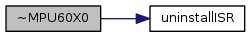## Member Function Documentation

 bool init ( )

set up initial values and start operation

Returns
true if successful

Here is the call graph for this function: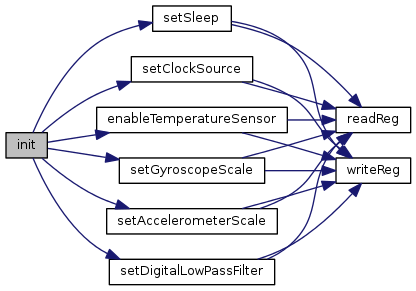Here is the caller graph for this function: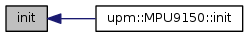void update ( void )

take a measurement and store the current sensor values internally. Note, these user facing registers are only updated from the internal device sensor values when the i2c serial traffic is 'idle'. So, if you are reading the values too fast, the bus may never be idle, and you will just end up reading the same values over and over.

Unfortunately, it is is not clear how long 'idle' actually means, so if you see this behavior, reduce the rate at which you are calling update().

Here is the call graph for this function: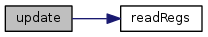Here is the caller graph for this function: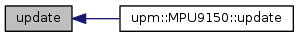uint8_t readReg ( uint8_t reg )

Parameters
Returns
the value of the register

Here is the caller graph for this function: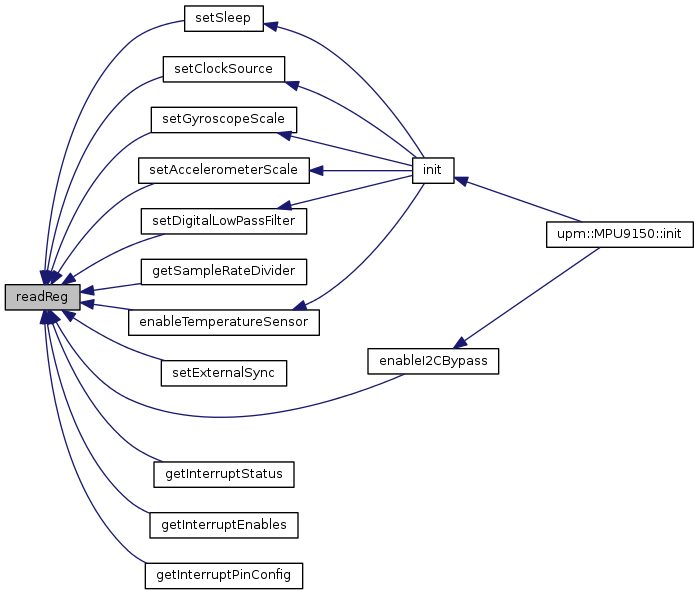void readRegs ( uint8_t reg, uint8_t * buffer, int len )

read contiguous refister into a buffer

Parameters
 reg the register to start reading at buffer the buffer to store the results len the number of registers to read
Returns
the value of the register

Here is the caller graph for this function: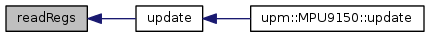bool writeReg ( uint8_t reg, uint8_t val )

write to a register

Parameters
 reg the register to write to val the value to write
Returns
true if successful, false otherwise

Here is the caller graph for this function: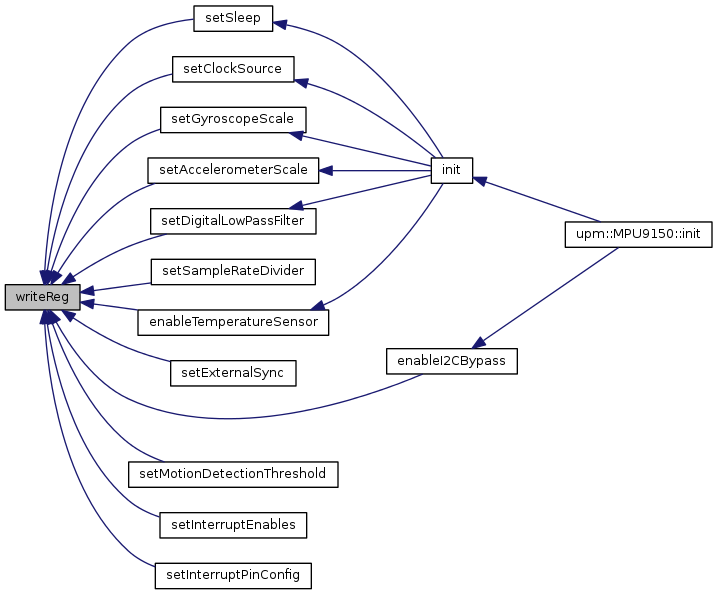bool setSleep ( bool enable )

enable or disable device sleep

Parameters
 enable true to put device to sleep, false to wake up
Returns
true if successful, false otherwise

Here is the call graph for this function: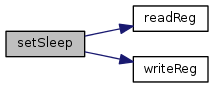Here is the caller graph for this function:bool setClockSource ( CLKSEL_T clk )

specify the clock source for the device to use

Parameters
 clk one of the CLKSEL_T values
Returns
true if successful, false otherwise

Here is the call graph for this function: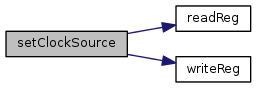Here is the caller graph for this function: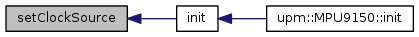bool setGyroscopeScale ( FS_SEL_T scale )

set the scaling mode of the gyroscope

Parameters
 scale one of the FS_SEL_T values
Returns
true if successful, false otherwise

Here is the call graph for this function: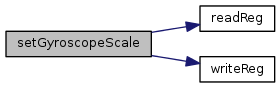Here is the caller graph for this function: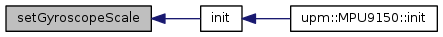bool setAccelerometerScale ( AFS_SEL_T scale )

set the scaling mode of the accelerometer

Parameters
 scale one of the AFS_SEL_T values
Returns
true if successful, false otherwise

Here is the call graph for this function: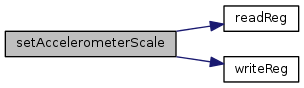Here is the caller graph for this function: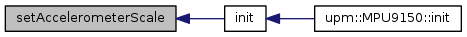bool setDigitalLowPassFilter ( DLPF_CFG_T dlp )

set the Low Pass Digital filter. This enables filtering (if non-0) of the accelerometer and gyro outputs.

Parameters
 dlp one of the DLPF_CFG_T values
Returns
true if successful, false otherwise

Here is the call graph for this function: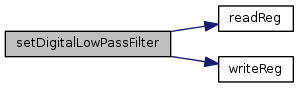Here is the caller graph for this function: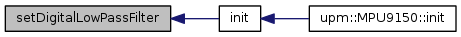bool setSampleRateDivider ( uint8_t div )

set the sample rate divider. This register specifies the divider from the gyro output rate used to generate the Sample Rate. The sensor registor output, FIFO output, DMP sampling and motion detection are all based on the Sample Rate.

The Sample Rate is generated by dividing the gyro output rate by this register:

Sample Rate = Gyro output rate / (1 + sample rate divider).

The Gyro output rate is 8Khz when the Digital Low Pass Filter (DLPF) is 0 or 7 (DLPF_260_256 or DLPF_RESERVED), and 1Khz otherwise.

Parameters
 div one of the DLPF_CFG_T values
Returns
true if successful, false otherwise

Here is the call graph for this function: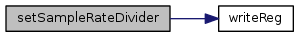uint8_t getSampleRateDivider ( )

get the current Sample Rate divider

Returns
the current sample rate divider

Here is the call graph for this function: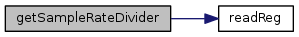void getAccelerometer ( float * x, float * y, float * z )

get the accelerometer values

Parameters
 x the returned x value, if arg is non-NULL y the returned y value, if arg is non-NULL z the returned z value, if arg is non-NULL
Returns
true if successful, false otherwise
 void getGyroscope ( float * x, float * y, float * z )

get the gyroscope values

Parameters
 x the returned x value, if arg is non-NULL y the returned y value, if arg is non-NULL z the returned z value, if arg is non-NULL
Returns
true if successful, false otherwise
 float getTemperature ( void )
virtual

get the temperature value

Returns
the temperature value in degrees Celsius

Reimplemented in MPU9250.

 bool enableTemperatureSensor ( bool enable )

enable onboard temperature measurement sensor

Parameters
 enable true to enable temperature sensor, false to disable
Returns
true if successful, false otherwise

Here is the call graph for this function: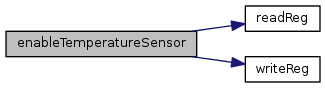Here is the caller graph for this function: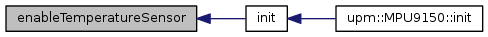bool setExternalSync ( EXT_SYNC_SET_T val )

configure external sync. An external signal connected to the FSYNC pin can be sampled by configuring EXT_SYNC_SET. Signal changes to the FSYNC pin are latched so that short strobes may be captured. The latched FSYNC signal will be sampled at the Sampling Rate, as defined in register 25. After sampling, the latch will reset to the current FSYNC signal state.

The sampled value will be reported in place of the least significant bit in a sensor data register determined by the value of EXT_SYNC_SET

Parameters
 val one of the EXT_SYNC_SET_T values
Returns
true if successful, false otherwise

Here is the call graph for this function: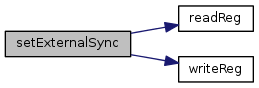bool enableI2CBypass ( bool enable )

enable I2C Bypass. Enabling this feature allows devices on the MPU60X0 auxiliary I2C bus to be visible on the MCU's I2C bus.

Parameters
 enable true to I2C bypass
Returns
true if successful, false otherwise

Here is the call graph for this function: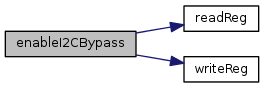Here is the caller graph for this function: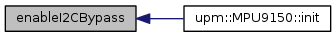bool setMotionDetectionThreshold ( uint8_t thr )

set the motion detection threshold for interrupt generation. Motion is detected when the absolute value of any of the accelerometer measurements exceeds this Motion detection threshold.

Parameters
 thr threshold
Returns
true if successful, false otherwise

Here is the call graph for this function:uint8_t getInterruptStatus ( )

return the interrupt status register.

Returns
the interrupt status word (see INT_STATUS_BITS_T)

Here is the call graph for this function: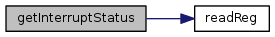bool setInterruptEnables ( uint8_t enables )

set the interrupt enables

Parameters
 enables bitmask of INT_ENABLE_BITS_T values to enable
Returns
true if successful, false otherwise

Here is the call graph for this function: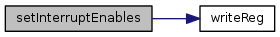uint8_t getInterruptEnables ( )

get the current interrupt enables register

Returns

Here is the call graph for this function: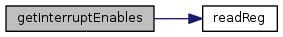bool setInterruptPinConfig ( uint8_t cfg )

set the interrupt pin configuration

Parameters
Returns
true if successful, false otherwise

Here is the call graph for this function: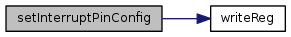uint8_t getInterruptPinConfig ( )

get the current interrupt pin configuration

Returns

Here is the call graph for this function: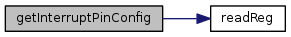void installISR ( int gpio, mraa::Edge level, void(*)(void *) isr, void * arg )

install an interrupt handler.

Parameters
 gpio gpio pin to use as interrupt pin level the interrupt trigger level (one of mraa::Edge values). Make sure that you have configured the interrupt pin (setInterruptPinConfig()) properly for whatever level you choose. isr the interrupt handler, accepting a void * argument arg the argument to pass the the interrupt handler

Here is the call graph for this function: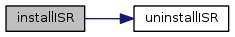void uninstallISR ( )

uninstall a previously installed interrupt handler

Here is the caller graph for this function: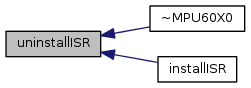Inheritance diagram for MPU60X0: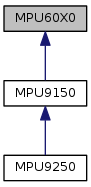[legend]

The documentation for this class was generated from the following files: